### IMO Shortlist 1995 problem S3

Kvaliteta:
Avg: 0,0
Težina:
Avg: 7,0
For an integer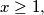$x \geq 1,$ let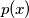$p(x)$ be the least prime that does not divide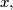$x,$ and define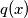$q(x)$ to be the product of all primes less than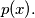$p(x).$ In particular,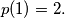$p(1) = 2.$ For$x$ having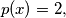$p(x) = 2,$ define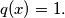$q(x) = 1.$ Consider the sequence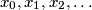$x_0, x_1, x_2, \ldots$ defined by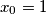$x_0 = 1$ and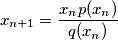for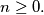$n \geq 0.$ Find all$n$ such that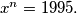$x^n = 1995.$
Izvor: Međunarodna matematička olimpijada, shortlist 1995